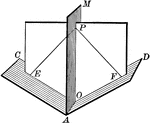### Dihedral Angle With Bisector

Illustration of a dihedral angle bisected by a plane. "Every point in a plane which bisects a dihedral…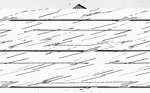### Dihedral Angle

Illustration of dihedral angle AB (or M-AB-N) formed by planes.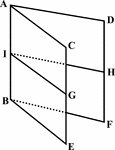### Dihedral Angle

Illustration of dihedral angle AB formed by planes.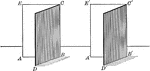### Two Equal Dihedral Angles

Illustration of 2 equal dihedral angles.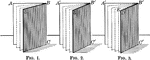### Equal Dihedral Angles

Illustration of 3 equal dihedral angles. "Two dihedral angles have the same ratio as their plane angles."